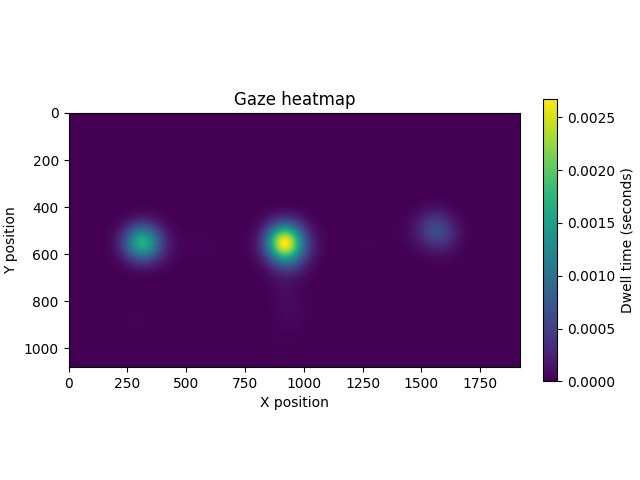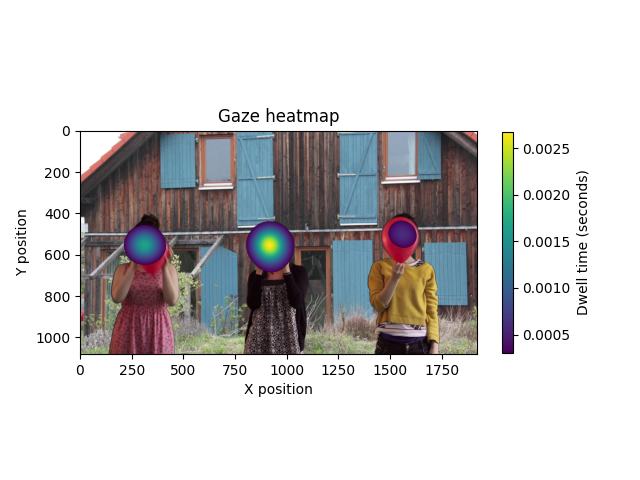# Plotting eye-tracking heatmaps in MNE-Python#

This tutorial covers plotting eye-tracking position data as a heatmap.

```# License: BSD-3-Clause
```

As usual we start by importing the modules we need and loading some example data: eye-tracking data recorded from SR research’s `'.asc'` file format.

```import matplotlib.pyplot as plt

import mne
from mne.viz.eyetracking import plot_gaze

stim_fpath = task_fpath / "stim" / "naturalistic.png"

```
```Loading /home/circleci/mne_data/MNE-eyelink-data/freeviewing/sub-01_task-freeview_eyetrack.asc
Pixel coordinate data detected.Pass `scalings=dict(eyegaze=1e3)` when using plot method to make traces more legible.
Pupil-size area detected.
There are 10 recording blocks in this file. Times between blocks will be annotated with BAD_ACQ_SKIP.
```

## Process and epoch the data#

First we will interpolate missing data during blinks and epoch the data.

```mne.preprocessing.eyetracking.interpolate_blinks(raw, interpolate_gaze=True)
raw.annotations.rename({"dvns": "natural"})  # more intuitive
event_ids = {"natural": 1}
events, event_dict = mne.events_from_annotations(raw, event_id=event_ids)

epochs = mne.Epochs(
raw, events=events, event_id=event_dict, tmin=0, tmax=20, baseline=None
)
```
```Interpolating missing data during blinks...
Used Annotations descriptions: ['natural']
5 matching events found
No baseline correction applied
0 projection items activated
```

## Plot a heatmap of the eye-tracking data#

To make a heatmap of the eye-tracking data, we can use the function `plot_gaze()`. We will need to define the dimensions of our canvas; for this file, the eye position data are reported in pixels, so we’ll use the screen resolution of the participant screen (1920x1080) as the width and height. We can also use the sigma parameter to smooth the plot.

```px_width, px_height = 1920, 1080
cmap = plt.get_cmap("viridis")
plot_gaze(epochs["natural"], width=px_width, height=px_height, cmap=cmap, sigma=50)
``````Using data from preloaded Raw for 5 events and 10001 original time points ...
```

## Overlaying plots with images#

We can use matplotlib to plot gaze heatmaps on top of stimuli images. We’ll customize a `Colormap` to make some values of the heatmap completely transparent. We’ll then use the `vlim` parameter to force the heatmap to start at a value greater than the darkest value in our previous heatmap, which will make the darkest colors of the heatmap transparent.

```cmap.set_under("k", alpha=0)  # make the lowest values transparent
ax = plt.subplot()
plot_gaze(
epochs["natural"],
width=px_width,
height=px_height,
vlim=(0.0003, None),
sigma=50,
cmap=cmap,
axes=ax,
)
``````Using data from preloaded Raw for 5 events and 10001 original time points ...